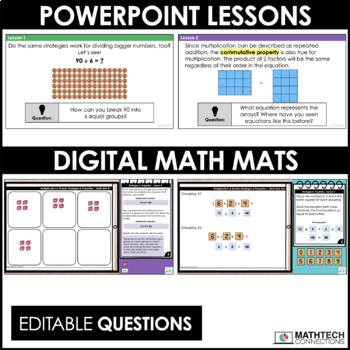# 3rd Grade Multiplication & Division Strategies Properties Guided Math Curriculum3rd
Subjects
Standards
Resource Type
Formats Included
• Zip
•Google Apps™
Pages
230+The Teacher-Author indicated this resource includes assets from Google Workspace (e.g. docs, slides, etc.).
##### Also included in
1. I’m so excited to share with you Unit 1 of our 3rd Grade Print &amp; Digital Guided Math Curriculum! Save 40% when you purchase Unit 1. If you purchase individually, this unit has a value of \$102.This unit includes 34 individual lessons with hands-on activities and independent practice - both print
Price \$61.20Original Price \$102.00Save \$40.80
2. Save \$189 when you purchase this complete bundle!Our 3rd Grade Guided Math Curriculum includes 126 editable step-by-step lessons! This curriculum provides 5 major components of a workshop model: Step-by Step Math Mini-Lessons, hands-on activities, independent practice, exit tickets, and assessments.
Price \$189.00Original Price \$378.00Save \$189.00

### Description

This Multiplication and Division Strategies and Properties Mini-unit includes both print & digital resources. It is designed with guided math workshop in mind and includes the following:

Lessons Included:

• 5.1 Hands-on problem solving within 100
• 5.2 Commutative property
• 5.3 Associative property
• 5.4 Distributive property
• 5.5 Order of operations

EACH LESSON INCLUDES THE FOLLOWING:

• PowerPoint Lesson: Bright, engaging digital lessons introduce and model each concept, including interactive questions and error analysis tasks to check understanding. (editable text)

• Math Mat & Task Cards: Task cards guide students through hands-on activities designed to reinforce the concepts introduced in the Mini-Lesson. (editable questions)

• Practice Pages: Perfect for in-class practice or homework, these activities provide plenty of support, but ask students to start demonstrating their understanding more independently.

• Exit Tickets provide a brief snapshot of student understanding for you at the end of each math block. (editable text)

ALSO INCLUDED:

• Quick Check (lessons 1-5 quiz with editable questions)
• All digital options

CCSS: 3.OA.5, 3.OA.7, 3.OA.8

TEKs: 3.4G

SAVE BIG:

Total Pages
230+
Included
Teaching Duration
N/A
Report this resource to TpT
Reported resources will be reviewed by our team. Report this resource to let us know if this resource violates TpT’s content guidelines.

### Standards

to see state-specific standards (only available in the US).
Apply properties of operations as strategies to multiply and divide. Examples: If 6 × 4 = 24 is known, then 4 × 6 = 24 is also known. (Commutative property of multiplication.) 3 × 5 × 2 can be found by 3 × 5 = 15, then 15 × 2 = 30, or by 5 × 2 = 10, then 3 × 10 = 30. (Associative property of multiplication.) Knowing that 8 × 5 = 40 and 8 × 2 = 16, one can find 8 × 7 as 8 × (5 + 2) = (8 × 5) + (8 × 2) = 40 + 16 = 56. (Distributive property.)
Fluently multiply and divide within 100, using strategies such as the relationship between multiplication and division (e.g., knowing that 8 × 5 = 40, one knows 40 ÷ 5 = 8) or properties of operations. By the end of Grade 3, know from memory all products of two one-digit numbers.
Solve two-step word problems using the four operations. Represent these problems using equations with a letter standing for the unknown quantity. Assess the reasonableness of answers using mental computation and estimation strategies including rounding.

### Questions & Answers

Teachers Pay Teachers is an online marketplace where teachers buy and sell original educational materials.